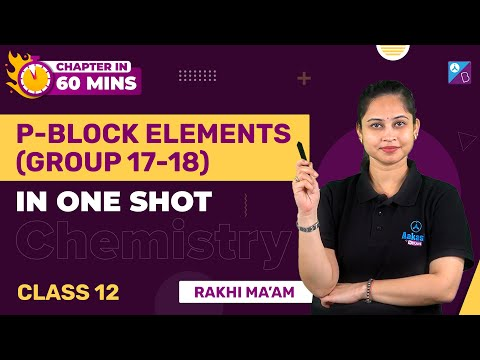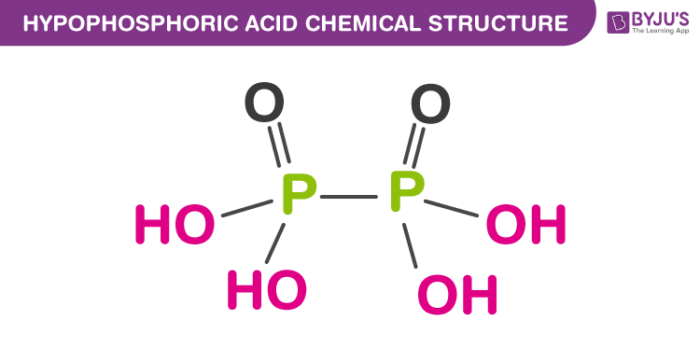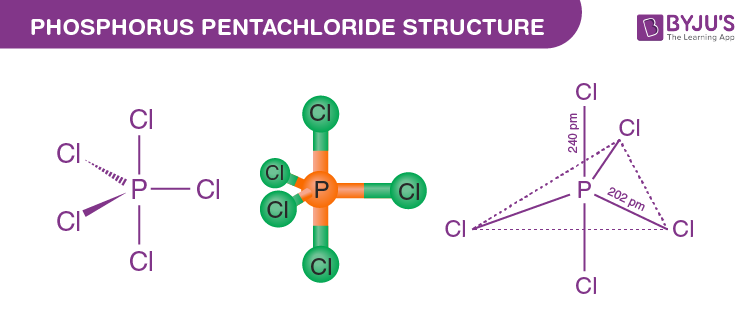# Important Questions for Class 12 Chemistry Chapter 7 – P – Block Elements

Class 12 chemistry important questions with answers are provided here for chapter 7 P – Block Elements. These important questions are based on the CBSE board curriculum and correspond to the most recent Class 12 chemistry syllabus. By practising these Class 12 important questions, students will be able to quickly review all of the ideas covered in the chapter and prepare for the Class 12 Annual examinations as well as other entrance exams such as NEET and JEE.

Download Class 12 Chemistry Chapter 7 – P – Block Elements Important Questions with Answers PDF by clicking on the button below.

## p-Block Elements Chemistry One Shot## Class 12 P – Block Elements Important Questions with Answers

Q1. In the preparation of H2SO4 by Contact Process, why is SO3 not absorbed directly in water

to form H2SO4?

SO3 is not absorbed directly in water to form H2SO4 because SO3 forms a dense fog of sulphuric acid which does not condense easily.

Q2. Write a balanced chemical equation for the reaction showing catalytic oxidation of NH3 by

atmospheric oxygen.

The balanced chemical equation for the reaction catalytic oxidation of NH3 by atmospheric oxygen is

$$\begin{array}{l}4NH_{3}+5O_{2}\xrightarrow[500K]{Pt/Rauge\ catalyst}4NO+6H_{2}O\end{array}$$

Q3. Write the structure of hypophosphoric acid.Q4. PH3 forms bubbles when passed slowly in water, but NH3 dissolves. Explain why?

PH3 forms bubbles because it is insoluble in water and cannot form hydrogen bonds with water, whereas NH3 dissolves because it is soluble in water and can form hydrogen bonds with water.

Q5. In PCI5, phosphorus is in a sp3d hybridised state, but its five bonds are not equivalent.

PCI5 has a trigonal bipyramidal structure. It has three equivalent equatorial bonds and two equivalent axial bonds. The size of axial bonds is greater than equatorial bonds to overcome repulsion because the three equatorial bonds cause more repulsion.Therefore two axial P-Cl bonds are more prolonged and different from equatorial bonds.

Q6. Why is nitric oxide paramagnetic in a gaseous state, but the solid obtained on cooling it is

diamagnetic?

NO2 exists as a monomer with one unpaired electron in a gaseous state. In the solid state, it dimerises to N2O4 , so no unpaired electron is left; hence solid form is diamagnetic.

Q7. Give reason to explain why CIF3 exists but FCl3 does not exist.

Chlorine has vacant d orbitals; hence it can show an oxidation state of +3. Fluorine has no d orbitals; it cannot exhibit a positive oxidation state. Thus, FCl3 does not exist.

The concept of atomic size can also explain it. The size of the chlorine atom is more significant. Small size fluorine atoms cannot accommodate three large chlorine atoms.

Thus, CIF3 exists, but FCl3 does not exist.

Q8. Out of H2O and H2S, which one has a higher bond angle and why?

H2O has a higher bond angle than H2S.

The bond angle of H2O is larger because oxygen is more electronegative than sulphur; therefore, the bond pair electron of the O−H bond will be closer to oxygen. Due to bond-pair bond-pair repulsion between bond pairs of two O−H bonds, the bond angle would be more prominent.

Q9. SF6 is known, but SCl6 is not. Why?

A fluorine atom is smaller, so six F ions can surround a sulphur atom. The case is not so with chlorine atoms due to their large size. So, SF6 is known, but SCl6 is not known due to interionic repulsion between larger ions.

Q10. In reaction with Cl2, phosphorus forms two halides, ‘A’ and ‘B’. Halide A is

yellowish-white powder, but halide “B’ is a colourless oily liquid. Identify A and B and write the

formulas of their hydrolysis products.

A is PCl5 (Yellowish-white powder)

P4 + 10Cl2 → 4PCl5

B is PCl3 (Colourless Oily liquid)

P4 + 6Cl2 → 4PCl3

Hydrolysis products of A and B

PCl3 + 3H2O → H3PO3 + 3HCl

PCl5 + 4H2O → H3PO4 + 5HCl

Q11. In the ring test of NO3ion, Fe2+ ion reduces nitrate ion to nitric oxide, which combines

with Fe2+ (aq) ion to form a brown complex. Write the reactions involved in the formation

of the brown ring.

NO3 + 3Fe2+ + 4H+ → NO + 3Fe3+ + 2H2O

[Fe(H2O)6]2+ + NO → [Fe(H2O)5(NO)]2+ + H2O

Q12. Explain why the stability of oxyacids of chlorine increases in the order given below: HCIO < HCIO2 < HCIO3 < HCIO4.

Oxygen is more electronegative than chlorine. Therefore dispersal of negative charge present on chlorine increases from ClO to ClO4 ion because the number of oxygen atoms attached to chlorine increases. Therefore, the stability of ions will increase in the order given below.

ClO< ClO2< ClO3< ClO4

Due to the increased stability of the conjugate base, the acidic strength of the corresponding acid rises in the same order.

HCIO < HCIO2 < HCIO3 < HCIO4.

Q13. Explain why ozone is thermodynamically less stable than oxygen.

Ozone is thermodynamically unstable concerning oxygen since its decomposition into oxygen results in the liberation of heat (ΔH is negative) and increased entropy ( ΔS is positive). These two effects reinforce each other, resulting in significant negative Gibbs energy change ( ΔG) for its conversion into oxygen.

Q14. P4O6 reacts with water according to the equation P4O6 + 6H2O → 4H3PO3. Calculate the volume of 0.1MNaOH solution required to neutralise the acid formed by dissolving 1.1g of P4O6 in H2O.

P4O6 + 6H2O → 4H3PO3 … (i)

For neutralisation

4× [H3PO3 + 2NaOH → Na2HPO3 + 2H2O] … (ii)

P4O6 + 8NaOH → 4Na2HPO3 + 2H2O

P4O6 (mol. mass) = (4×31+16×6) = 220

The number of moles of P4O6 = Given mass / Molar Mass.

= 1.1 / 220

∵ The product formed by 1.1 / 220 of P4O6 will be neutralised by 8 moles of NaOH.

∴ P4O6 = 8 × 1.1 / 220 = 8.8 / 220 mol NaOH

Given molarity of NaOH in 1L = 0.1M

Molarity = No. of Moles / Volume in litres

Volume= No. of Moles / Molarity

= 8.8 / 220 × 1 / 0.1

=0.4L

Q15. White phosphorus reacts with chlorine, and the product hydrolyses in the presence of

water. Calculate the mass of HCl obtained by the hydrolysis of the product formed by the

reaction of 62 g of white phosphorus with chlorine in the presence of water.

P4 + 6Cl2 → 4PCl3

[ PCl3 + 3H2O → H3PO3 + 3HCl ] × 4

P4 + 6Cl2 + 12H2O → 4H3PO3 + 12HCl

1 mol of white phosphorus produces 12 moles of HCl

62 g of white phosphorus has been taken, which is equivalent to 62 / 124 = 1 / 2 mol

Therefore 6 moles of HCl will be formed.

Mass of 6 moles of HCl = 6 × 36.5 = 219.0 g of HCl

Q16. Name three oxoacids of nitrogen. Write the disproportionation reaction of that oxoacid of

nitrogen in which nitrogen is in +3 oxidation state.

The three oxoacids of nitrogen are

(a) Nitrous acid, HNO2

(b) Nitric acid, HNO3

(c) Hyponitrous acid, H2N2O2Q17. Nitric acid forms an oxide of nitrogen on reaction with P4O10. Write the reaction involved.

Nitric acid reacts with P4O10 to form an oxide of nitrogen, N2O5 and metaphosphoric acid, HPO3.

The reaction follows

HNO3 + P4O10 → 4HPO3 + 2N2O5 .

Q18. Phosphorus has three allotropic forms- (A) White phosphorus, (B) Red phosphorus and (C) Black phosphorus. Write the difference between white and red phosphorus based on their structure and reactivity.

Property White Phosphorous Red Phosphorous
Colour White Phosphorous is white, but on exposure, it turns yellow. Red phosphorous is dark red in colour.
State It is a waxy solid. It is a brittle powder.
Density Its density is 1.84 g/cm3. Its density is 2.1 g/cm3.
Ignition temperature Its ignition temperature is 307 K. Its ignition temperature is 543 K.
Chemical Reactivity It is highly reactive It is less reactive.

Q19. Give an example to show the effect of nitric acid concentration on the formation of

an oxidation product.

Dilute and concentrated nitric acid give different oxidation products on reaction with copper metal.

3Cu + 8HNO3 (dil.) → 3Cu(NO3)2 + 2NO + 4H2O

Cu + 4HNO3 (Conc) → 3Cu(NO3)2 + 2NO2 + 2H2O

Q20. PCI5 reacts with finely divided silver on heating, and a white silver salt is obtained, which

dissolves on adding excess aqueous NH3 solution. Write the reactions involved to explain

what happens.

PCl5 + 2Ag → 2AgCl + PCl3

AgCl + 2NH3 (aq) → [Ag(NH3)2] + Cl

Q21. Phosphorus forms several oxoacids. Out of these oxoacids, phosphinic acid has

strong reducing property. Write a reaction showing its reducing

behaviour.

The reducing behaviour of phosphinic acid is observable in the reaction with silver nitrate given below:

4AgNO3 + 2H2O + H3PO2 → 4Ag + 4HNO3 + H3PO4

Q1. An amorphous solid A burns in air to form a gas B which turns lime water milky. The gas is also produced as a by-product during the roasting of sulphide ore. This gas decolourises acidified aqueous KMnO4 solution and reduces Fe3+ to Fe2+. Identify the solid A and the gas B.

(i) Since gas ‘B’ is obtained as a by-product during roasting of sulphide, therefore, gas ‘B’ must be Sulphur dioxide, SO2.

2ZnS + 3O2 → 2ZnO + 2SO2 (B)

(ii) Since gas ‘B’ is obtained when amorphous solid ‘A’ burns in air, therefore, amorphous solid ‘A’ must be sulphur, S8.

S8 (A) + 8 O → 8S2 (B)

Thus, solid′A′= Sulphur S8 and gas ′B′= Sulphur dioxide (SO2).

Q2. On heating lead (II) nitrate gives a brown gas ” A”. The gas ” A” on cooling changes to colourless solid “B” . Solid B on heating with NO changes to a blue solid C . Identify ‘ A’, ‘B’ and C and also write reactions involved and draw the structures of ‘B’ and ‘C’ .

(i) Since lead (II) nitrate on heating gives a brown gas ‘A’ therefore, gas ‘A’ must be nitrogen dioxide (NO2).

2Pb(NO3) → 2PbO + 4NO2 + O2

(ii) The brown gas ‘A’ on cooling dimerises to give a colourless solid ‘B’; therefore ‘, B’ must be N2O4 (dinitrogen tetroxide).

2NO2 ↔ N2O4

(iii) Since colourless solid ‘B’ on heating with NO gives a blue solid ‘C’ therefore ‘, C must be dinitrogen trioxide.

2NO + N2O4 → 2N2O3

Thus, A = NO2, B = N2O4 and C = N2O3.

Q3.On heating, compound A gives a gas B, a constituent of air. When treated with 3mol of hydrogen (H2) in the presence of a catalyst, this gas provides another gas with C, which is basic. Gas C on further oxidation in moist conditions gives a compound D part of acid rain. Identify compounds A to D.

Give the necessary equations of all the steps.

Here,

A = NH4NO2

B = N2

C = NH3

D = HNO3

The necessary equations are:

NH4NO2 → N2 + 2H2O

N2 + 3H2 → 2NH3

4NH3 + 5O2 → 4NO + 6H2O

4NO + O2 → 4NO2

3NO2 + H2O → 2HNO3 + NO

Test your Knowledge on Important questions class 12 chemistry chapter 7 p-block elements!